×
Get Full Access to The U - OIS 2340 - Study Guide - Midterm
Get Full Access to The U - OIS 2340 - Study Guide - Midterm

×

THE U / Information System / OIS 2340 / What are the labels or names used to identify an element?

# What are the labels or names used to identify an element? Description

##### Description: So I know our class is really confusing but I've been reading the book and going to tutoring. Theres a file in canvas called "Equation Sheet" basically if you understand all those equations and can do them you'll be set, and we'll have that sheet on the test. So this study guide breaks down each of the equations and explains them with some examples.
9 Pages 76 Views 4 Unlocks
Reviews

Chapter 1

## What are the labels or names used to identify an element?Types of Data (levels of measurement)● The Higher the data level the more sophisticated analysis you can perform Nominal: Labels or names used to identify an element, a non numeric label or a numeric code may be used. (ex. Hair color, marital status, could you m for married for s for single)

Ordinal: have the properties of nominal data but the order or rank of the data matters. A non numeric label or numeric code may be used. (ex. Freshman, Sophomore, Junior, Senior) - Likert Scale Data: Rate your experience on a scale from 1-10

## What has the properties of nominal data but the order or rank of the data matter?- Ordinal data should NOT be multiplied

Interval: Have properties of ordinal data, with an interval between observations expressed in a fixed measurement. Always numeric (ex. Temperature scale)

Ratio: has the property of interval data and the ratio of two values is MEANINGFUL. Must have a zero scale to represent that nothing exists at zero (ex. Distance, weight, time. Money in your bank account vs money in your parent's bank account)

Chapter 2

Difference between histogram and bar chart Don't forget about the age old question of What is a paramecium?

- No spaces

- Bar chart has categories as one axis and numerical values on the other axis, Histogram has two numerical values on both axis.

## What has properties of ordinal data, with an interval between observations expressed in a fixed measurement?- Histogram has interval or ratio data

Frequency distribution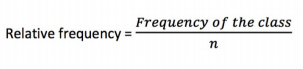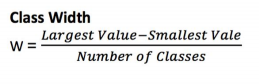Chapter 3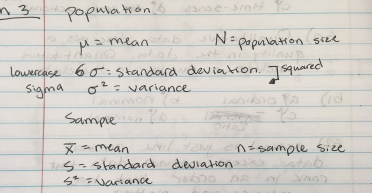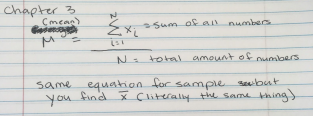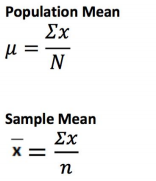If you want to learn more check out What are the examples of baroque?
We also discuss several other topics like What is the meaning of enantiomers?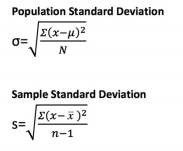** Notice that when taking the sample you divide by the total amount of numbers minus 1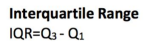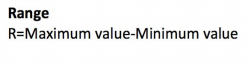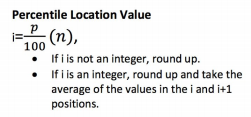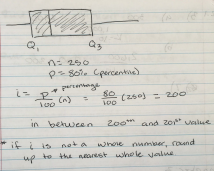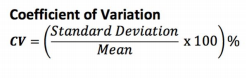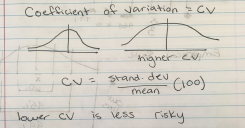If you want to learn more check out How does a modem work?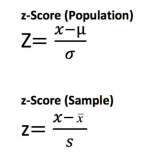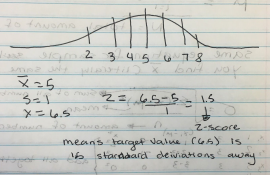This is saying how many standard deviations away the numbers are If you want to learn more check out How did the work of barry schwartz and allen neuringer support or advance the understanding of response stereotypes and response variability?
If you want to learn more check out Gregory gets very emotional while watching sad romance movies and normally cries during them. which archetype is he displaying?

Weighted Mean: mean of data values that have been weighted according to their relative importance

Xi = value of observation (the ith data value)

Wi = Weight for observation i

Calculating a weighted mean

1. Collect the desired data and determine the weight to be assigned to each data value (ex. If you take all the assignments from a class and then list the points possible and the points you actually got)

Points Possible

150

100

50

50

200

250

140

100

50

25

150

200

2. Multiply each weight by the data value and add them all together. = (140)(150) + (100)(100) + (50)(50) + (25)(50) + (150)(200) + (250)(200) = 115500

3. Sum the weights for all values (what you received on the assignment)

= 140 +100 + 50 +25 +150 +200 = 665

4. Compute the weighted Mean.

- Divide the weighted sum by sum of the weights

115500 ÷665 = 173.68

Chapter 4

Chapter 5

Means that you know all of your probabilities and they all add up to one

q= 1 - p

n = Sample Size

T = Sample Size ÷ Segment size

Page ExpiredIt looks like your free minutes have expired! Lucky for you we have all the content you need, just sign up here